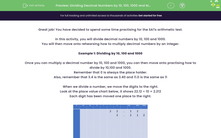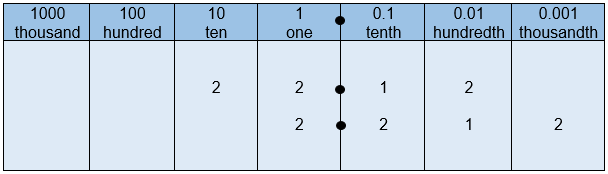# Dividing Decimal Numbers by 10, 100, 1000 and Multiplying by an Integer

In this worksheet, students will rehearse how to divide decimal numbers by 10, 100 and 1000. They will also practice how to multiply decimals by integers. These place value skills need to be cemented for the SATs Arithmetic paper.This content is premium and exclusive to EdPlace subscribers.Key stage:  KS 2

Curriculum topic:   Exam-style Questions: SATs Maths

Curriculum subtopic:   Exam-Style Questions: Accurate Decimal Calculations

Difficulty level:#### Worksheet Overview

Great job! You have decided to spend some time practising for the SATs arithmetic test.

In this activity, you will divide decimal numbers by 10, 100 and 1000.

You will then move onto rehearsing how to multiply decimal numbers by an integer.

Example 1: Dividing by 10, 100 and 1000

Once you can multiply a decimal number by 10, 100 and 1000, you can then move onto practising how to divide by 10,100 and 1000.

Remember that 0 is always the place holder.

Also, remember that 3.4 is the same as 3.40 and 11.0 is the same as 11

When we divide a number, we move the digits to the right.

Look at the place value chart below, it shows 22.12 ÷ 10 = 2.212

Each digit has been moved one place to the right.22.12 ÷ 10 = 2.212....All of the digits have moved one place to the right.

22.12 ÷  100 = 0.2212....All of the digits have moved two places to the right.

22.12 ÷ 1000 = 0.02212....All of the digits have moved three places to the right.

The decimal point does not move.

Example 2: Multiplying Decimals by Integers

When you multiply a decimal number by an integer, you may choose to use the column or grid method of multiplication.

Grid method:Here is the same calculation, solved using the column method:Now, over to you to try some of the practice questions...good luck!

### What is EdPlace?

We're your National Curriculum aligned online education content provider helping each child succeed in English, maths and science from year 1 to GCSE. With an EdPlace account you’ll be able to track and measure progress, helping each child achieve their best. We build confidence and attainment by personalising each child’s learning at a level that suits them.

Get started